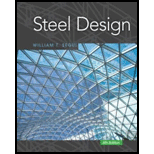Chapter 1, Problem 1.5.2P### Steel Design (Activate Learning wi...

6th Edition
Segui + 1 other
ISBN: 9781337094740

#### Solutions

Chapter
Section### Steel Design (Activate Learning wi...

6th Edition
Segui + 1 other
ISBN: 9781337094740
Textbook Problem

# A tensile test was performed on a metal specimen having a circular cross section with a diameter of 1 2 inch. The gage length (the length over which the elongation is measured) is 2 inches. For a load 13.5 kips, the elongation was 4.66 × 10 − 3 inches. If the load is assumed to be within the linear elastic rang: of the material, determine the modulus of elasticity.

To determine

The modulus of elasticity of metal specimen.

Explanation

Given:

The diameter of metal specimen is 12inch.

The gage length is 2inches.

The elongation is 4.66×103inches.

The load acting is 13.5kips.

Concept Used:

Write the equation for Hook’s law.

σ=EεE=σε ...... (I)

Here, the modulus of elasticity is E, tensile stress is σ, and the strain is ε.

Write the equation to calculate the tensile stress.

σ=PA ...... (II)

Here, load is P and the cross-sectional area is A.

Calculate the cross-sectional area.

A=π4×d2 ...... (III)

Here, the diameter of metal specimen is d.

Write the equation to calculate strain.

ε=eL ...... (IV)

Here, the elongation is e and the gage length is L.

Calculation:

Calculate the cross sectional area of the specimen.

Substitute 12inch for d in Equation (III).

A=π4×(0.5inch)2=0.7853inch24=0.1963inch2

Calculate the tensile stress

### Still sussing out bartleby?

Check out a sample textbook solution.

See a sample solution

#### The Solution to Your Study Problems

Bartleby provides explanations to thousands of textbook problems written by our experts, many with advanced degrees!

Get Started

## Additional Engineering Solutions

#### Explain why we need standards and codes.

Engineering Fundamentals: An Introduction to Engineering (MindTap Course List)

#### What capabilities should a wireless security toolkit include?

Principles of Information Security (MindTap Course List)

#### Convert P = 5.00 atm into Pa, bar, and psia.

Fundamentals of Chemical Engineering Thermodynamics (MindTap Course List)